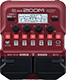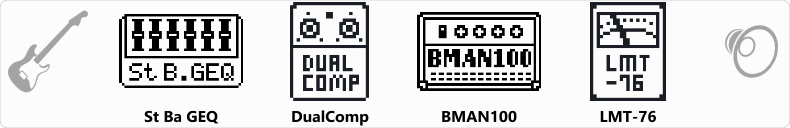# TEST nib black sabbath

Discussion in 'Zoom B1/B1X Four' started by riko, May 23, 2021.

1. TEST nib black sabbathDevice: Zoom B1 Four
Firmware: 1.10

Name on device: blk sbbth
Optimized for: Phones/Speaker

Effects chain:nib black sabbath intro test

Effect: "St Ba GEQ" (Filter), active - "yes"
"50" = 7.0
"120" = 5.0
"400" = 5.0
"500" = 4.0
"800" = 0.0
"4.5k" = 0.0
"10k" = 6.0
"VOL" = 100

Effect: "DualComp" (Dynamics), active - "yes"
"Frequency" = 1.5k
"LoCMP" = 25
"HiCMP" = 20
"Volume" = 100

Effect: "BMAN100" (Amp simulator), active - "yes"
"Bass" = 34
"MID-F" = 630Hz
"Middle" = 53
"Treble" = 63
"Gain" = 59
"Deep" = Off
"Volume" = 57

Effect: "LMT-76" (Dynamics), active - "yes"
"Input" = 23
"Ratio" = 4:1
"REL" = 35
"Output" = 40

Patch Volume: 110